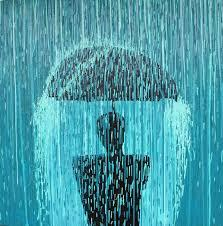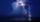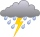# Rainfall

The annual average rainfall in India was in Cherrapunji in the year 1981 26 461 mm. How many hectoliters of water fell on 1 m2? Would fit this amount of water into a cube of three meters?

Result

V1 =  264.61 hl
y=

V1 =

### Step-by-step explanation:Did you find an error or inaccuracy? Feel free to write us. Thank you!Tips to related online calculators
Do you know the volume and unit volume, and want to convert volume units?

## Related math problems and questions:

• WaterOn the lawn which has area 914 m2 rained 3 mm of water. How many liters of water rained?
• Annual rainfallThe average annual rainfall is 686 mm. How many liters will fall on the 1-hectare field?
• RainfallA rectangular garden of 25m in length and width 20m in width fall 4mm of water. Express by a fraction in basic form what part of the 60-hectolitre tank we would fill with this water.
• RainfallOn Thursday, fell 1 cm rainfall. How many liters of water fell to rectangular garden with dimensions of 22 m x 35 m?
• Rainwater6 mm of water rained on the garden with an area of 25 acres. How many 12 liter cans of water would we water this garden just as much?
• Water lakeThe length of the lake water is 8 meters width 7 meters and depth 120 centimeters. How many liters of water can fit into the water lake?
• RainfallAnnual rainfall in our country is an average of 797 mm. How many m3 of water rains on average per hectare?
• Water tank 2Water tank cuboid is 12 meters long and 6.5 meters wide and 1.2 meters high. How many hectoliters are in the tank when it is filled to 81%?
• Cube and waterHow many liters of water can fit into a cube with an edge length of 0.11 m?
• The coilHow many ropes (the diameter 8 mm) fit on the coil (threads are wrapped close together) The coil has dimension: the inner diameter 400mm, the outside diameter 800mm and the length of the coil is 470mm
• RainfallHow many liters of water did fell in a 32m long and 8m wide garden, if 8mm of rain fell?
• How muchHow much money will we pay for 20 planks 4m long, 15cm wide and 26mm thick when 1m³ of wood costs 4500kč?
• Hectoliter into cubeCan 1hl of water fit into a half-meter cube?
• Water wellDrilled well has a depth of 20 meters and a 0.1 meters radius. How many liters of water can fit into the well?
• Water levelThe glass container has a cuboid shape with dimensions of the bottom 24 cm and 12 cm. The height of the water is 26 cm. Calculate the body's volume, which is sunk into the water if the water level rise by 3 cm.
• Truncated pyramidHow many cubic meters is the volume of a regular four-sided truncated pyramid with edges one meter and 60 cm and high 250 mm?
• CubeCalculate the surface of the cube ABCDA'B'C'D' if the area of rectangle ACC'A' = 344 mm2.